## LetsPlayMaths.Com

WELCOME TO THE WORLD OF MATHEMATICS

# Class-8 Rhombus

Introduction to Rhombus

Properties of Rhombus

Rhombus MCQ

Rhombus Worksheets

## Introduction to Rhombus

A parallelogram having all sides equal is known as rhombus. In the below given figure ABCD is a parallelogram having all sides equal i.e. AB = BC = CD = AD, hence we can conclude that ABCD is a rhombus.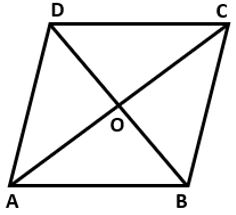## Properties of Rhombus

1. Opposite sides are parallel i.e., AB || CD and BC || AD
2. All sides are equal i.e., AB = BC = CD = AD
3. Opposite angles are equal i.e., ∠A = ∠C and ∠B = ∠D
4. Diagonals bisect each other i.e., AO = OC and BO = OD
5. Diagonals of a rhombus bisect each other at right angles i.e., ∠AOD = ∠COD = ∠AOB = ∠BOC = 90°

Example 1. Find the value of a, b and c in the below given rhombus.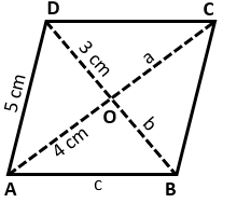Solution. Since all sides of a rhombus are equal.

AB = BC = CD = AD = 5 cm

=> c = 5 cm

Diagonals of a rhombus bisects each other.

AO = CO = 4 cm

a = 4 cm

DO = BO = 3 cm

b = 3 cm

Hence, a = 4 cm, b = 3 cm and c = 5 cm.

Example 2. In the below given figure, ABCD is a rhombus. If ∠A = 60°, find ∠CBD.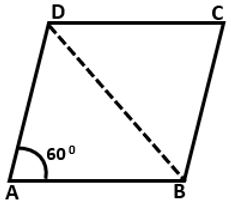Solution. Opposite angles of a rhombus are same.

∠A = ∠C = 60°

∠B = 180° − 60° (Adjacent angles of a rhombus are supplementary)

= 120°

∠CBD + ∠BDC + 60° = 180°

=> p° + p° + 60° = 180°

=> 2p° = 180° − 60°

=> 2p° = 120°

=> p° = 120°2

=> p° = 60°

Hence, ∠CBD = 60°.

Example 3. ABCD is a rhombus as shown in below given figure. Find the measure of ∠A.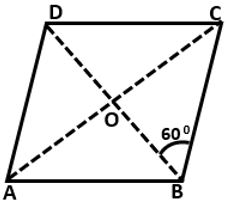Solution. ABCD is a rhombus and BCD is an isosceles triangle as BC = CD.

∠CBD = ∠BDC = 60°

∠CBD + ∠BDC + ∠BCD = 180° (Sum of all angles of a triangle = 180°)

=> 60° + 60° + ∠BCD = 180°

=> 120° + ∠BCD = 180°

=> ∠BCD = 180° − 120°

=> ∠BCD = 60°

=> ∠C = 60°

Opposite angles of a rhombus are equal

∠A = ∠C = 60°

Hence, ∠A = 60°.

Example 4. In the below given figure, ABCD is a rhombus. If ∠OCD = 30°, then find the measure of ∠OBC.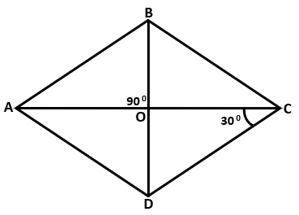Solution. ∠BAC = ∠ACD (AC is traversing through two parallel lines AB and CD)

∠BAC = 30°

Here ABC is an isosceles triangle where AB = BC = CD = AD

So, ∠BAC = ∠BCA = 30°

Here, OBC is a right-angle triangle.

∠OBC + ∠BOC + ∠BCO = 180° (Sum of all angles of a triangle = 180°)

=> ∠OBC + 90° + 30° = 180°

=> ∠OBC + 120° = 180°

=> ∠OBC = 180° − 120°

=> ∠OBC = 60°

Hence, ∠OBC = 60°.

Example 5. If the diagonals of a rhombus are 12 cm and 16 cm, find the length of each side.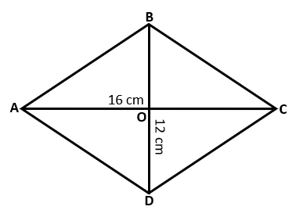Solution.Here ABCD is a rhombus, where AC = 16 cm and BD = 12 cm.

AO = CO = 8 cm (As we know rhombus diagonals bisects each other)

BO = DO = 6 cm

Rhombus diagonals bisect each other at an angle 90°. So, AOB is a right-angle triangle.

AB2 = AO2 + BO2 (As per Pythagoras theorem)

=> AB2 = 82 + 62

=> AB2 = 64 + 36

=> AB2 = 100

=> AB =  100

=> AB = 10 cm

Length of rhombus sides is equal to 10 cm.

Rhombus Test - 1

Rhombus Test - 2

## Class-8 Rhombus Worksheets

Rhombus Worksheet - 1

Rhombus Worksheet - 2